Next: Production:CDC_VTX;Track_Parameter Up: Banks in the Event Previous: Production:VTX;Space_Point

## Production:CDC;Track_Parameter


Element# 		: 		 Equal to the track number.
TBPUT by 		:		 Smearing routine.
Contents 		: 		 Smeared Charged track information.
Format 		: 		 R*4, R*8, I*2, and I*4 mixed.
variable length ( 56+number of contributed generator tracks.)



RBUF(1-3)=Í<>px, py, pz.
(4)=Í<>E assuming massless particle.
(5-7)=Í<>x, y, z of closest approach to the beam axis.
IBUF(9)=ápparent charge ( +/-1 )
RBUF(11-15)=d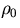(cm),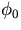(radian),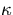(GeV-1), dZ(cm), and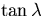of track parameter.
(16-18)=Pivot of track parameter, X0, Y0, andZ0.
DBUF(10-24)=érror matrix (lower triangle only)
RBUF(49)=not used ( reserved for confidence level )
(50)=not used ( reserved for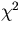)
HBUF(1,51)=Number of degrees of freedom.
(2,51)=not used ( reserved for duplicate flag )
(1,52)=Number of track parameters ( 5 for helices )
(2,52)=not used ( reserved for track finding type )
(1,53)=not used ( reserved for pointer to 1-st hit )
(2,53)=not used ( reserved for pointer to last hit )
RBUF(54-55)=radius of the innermost and the outmost hit
IBUF(56)=Number of contributing generator particle
(57)=Particle serial number of first generator particle.
.........

x, y, z, px, py, and pz in RBUF(1-7) are those at the closest approach point to the beam axis regardless of where the track actually started. On the other hand, (X0, Y0, Z0) in RBUF(16-18) is the innermost measured point in the CDC whose radius can be greater than the first wire layer radius for, for instance, a Ks which decayed in the midst of the CDC.Next: Production:CDC_VTX;Track_Parameter Up: Banks in the Event Previous: Production:VTX;Space_Point
Miyamoto Akiya
11/20/1998